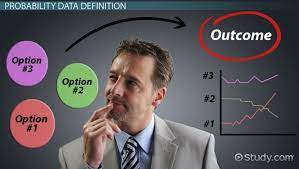In simple terms, probability is the likelihood of the occurrence of an event. There are various real-world situations that require us to make predictions. We may be certain or uncertain of the results of an event. In such scenarios, we say that there is a probability of the event to either occur or not occur. Probability has a wide range of applications in games, in business to make intelligent or guided speculations and even in artificial intelligence.

In order to calculate the probability of an event, we simply divide the favourable number of outcomes by the total number of likely outcomes. The value assigned to the probability of an event to occur can either be 0 or 1, since the favourable number of outcomes can never cross the total number of outcomes. Furthermore, the favourable number of outcomes cannot be negative. In the next paragraph, we will discuss in detail, the fundamentals of probability.

## What is Probability?

As mentioned earlier, probability is the ratio of the number of favourable outcomes to the total number of outcomes of an event. For instance, if ‘n’ represents the number of outcomes, and ‘x’, the number of favourable outcomes, the formula for deriving the probability of an event is as follows.

Probability (event) = Favourable outcomes / total outcomes = x/n

In order to understand this concept of probability better, we can use an example. A relatable illustration is a tossed coin, which can either drop on its head or tail, revealing the opposite side. Probability can also be used to predict the outcomes of events such as drawing a single card from a pack of playing cards or rolling a dice.

We can classify probability into theoretical and experimental.

## Common Theoretical Probability Terminologies

Here are some terms that are frequently used in probability.

Experiment: A trial or an operation conducted to produce an outcome is often referred to as an experiment.

Sample Space: All possible outcomes of an experiment are collectively known as a sample space. For example, the sample space of rolling a dice comprises all the numbers on each side of a side, from 1 to 6.

Favourable Outcome: The desired result or outcome produced by an event. For instance, if two dice are rolled, the favourable outcomes of getting the sum of numbers on the two dice as 5 are (2,3) and (4,1).

Trial: When a random experiment is performed, it is referred to as a trial.

Random Experiment: When a coin is tossed, we can either get a head or a tail. We call this a random experiment because the outcomes can not be predicted. Random experiments can be repeated under the same condition. The total number of outcomes of a random experiment is referred to as an event.

Event: The number of possible outcomes of an experiment is called an event.

Equally Likely Events: Applies to events that have the same theoretical probability or likelihood of occurring. For example, each numeral on a die is equally likely to occur when the die is tossed.

Exhaustive Events: We say an event is exhaustive when the set of all outcomes of an experiment matches the sample space.

Mutually Exclusive Events: These are events that are unable to occur simultaneously. A good example is the weather condition, it can either be wet or dry, cold or warm, but cannot be both.

In the next blog post, I will proceed to explain some of the common probability formulas and how they can be applied to real-life situations.## Transforming Nigeria’s Local Government System for Rural Economic Growth and Increased GDP

in## PROBABILITY – A TOOL FOR DECISION MAKING

in